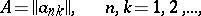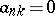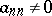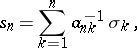# Triangular summation method

(diff) ← Older revision | Latest revision (diff) | Newer revision → (diff)

A matrix summation method defined by a triangular matrixthat is, by a matrix for whichfor. A triangulation summation method is a special case of a row-finite summation method. A triangular matrixis called normal iffor all. The transformationrealized by a normal triangular matrixhas an inverse:whereis the inverse of. This fact simplifies the proof of a number of theorems for matrix summation methods determined by normal triangular matrices. Related to the triangular summation methods are, e.g., the Cesàro summation methods and the Voronoi summation method.